﻿ 铝制高速双体船强度评估方法关键点研究
 舰船科学技术2017, Vol. 39Issue (2): 26-30PDF

1. 上海交通大学 海洋工程国家重点实验室, 上海 200240;
2. 高新船舶与深海开发装备协同创新中心, 上海 200240

Research on several critical points of the evaluation method for aluminum high-speed catamaran
XU Bo1,2, XIA Li-juan1,2
1. State Key Laboratory of Ocean Engineering, Shanghai Jiaotong University, Shanghai 200240, China;
2. Collaborative Innovation Center for Advanced Ship and Deep-Sea Exploration, Shanghai 200240, China
Abstract: In this paper, the evaluation method of the structure strength for the aluminum high-speed catamaran is discussed in detail. The influences to the strength for the aluminum high-speed catamaran of marine monitoring are analyzed and compared based on these two aspects:the calculation methods about the longitudinal bending moment distribution curve and applied positon of the total torque. Furthermore evaluations are achieved in the way of dangerous loading, and some recommendations are put forward. The related results have certain reference significance for structure design of similar vessels.
Key words: catamaran     structure strength     evaluation method     distribution curve     total torque
0 引 言

1 双体船结构强度评估有限元方法

 $\begin{split} & {M_{BY}} = 0.5(1 + \frac{{{a_{cg}}}}{g})(0.25L - \\ & S0.175\frac{\Delta }{{{B_s}d}}(1 + 0.2\frac{{{a_{cg}}}}{g}))\Delta g\text{。} \end{split}$ (1)

 ${M_{BX}} = 0.135\Delta {a_{cg}}b\text{，}$ (2)

 ${F_y} = \frac{{{M_{BX}}}}{{z + 0.5d}}\text{，}$ (3)

 ${M_{ty}} = 0.075\Delta {a_{cg}}L\text{。}$ (4)

 $p = \frac{{4{M_{ty}}}}{{{L^2}}}\text{。}$ (5)

2 若干关键点研究 2.1 总纵弯矩的分布曲线

 $\begin{split}\\[-12pt] & P(x) = \frac{{{M_{wo}}}}{{L_{ext}^2}}(20{\alpha _1}{x^3} + 12{\alpha _2}{x^2} - \\ & \quad \quad \quad \quad 6(2{\alpha _2} + 3{\alpha _1})x + 2(2{\alpha _1} + {\alpha _2})){\rm {kN/m}}\text{，} \end{split}$ (6)
 $\begin{split}\\[-12pt] & BM(x) = {M_{wo}}({\alpha _1}{x^5} + {\alpha _2}{x^4} - \\ & \quad \quad \quad \quad (2{\alpha _2} + 3{\alpha _1}){x^3} + (2{\alpha _1} + {\alpha _2}){x^2}){\rm {kN/m}}\text{。} \end{split}$ (7)

$x = \frac{d}{{{L_{ext}}}}$ $0 \leqslant x \leqslant 1$ d 为从尾封板到计算点的距离；

 \begin{aligned} {a_1} = & \frac{{{A_{22}} - {A_{12}}}}{{{A_{11}}{A_{22}} - {A_{21}}{A_{12}}}}\text{，}\\ {a_2} = & \frac{{{A_{21}} - {A_{11}}}}{{{A_{12}}{A_{21}} - {A_{11}}{A_{22}}}}\text{。} \end{aligned}

a1a2 计算中：

 \begin{aligned} {x_1} =& \frac{{\alpha + 0.4}}{{\alpha + \beta + 1}}\text{，}\\ {x_2} = & \frac{{\alpha + 0.65}}{{\alpha + \beta + 1}}\text{，}\\ {A_{11}} = & x_1^5 - 3x_1^3 + 2x_1^2\text{，}\\ {A_{22}} = & x_2^4 - 2x_2^3 + x_2^2\text{，}\\ {A_{12}} = & x_1^4 - 2x_1^3 + x_1^2\text{，}\\ {A_{21}} = & x_2^5 - 3x_2^3 + 2x_2^2\text{。} \end{aligned}

 $M(x) = {M_{BY}}\sin \frac{{\pi x}}{L}{ \rm {kN/m}}\text{，}$ (8)

Mx）可通过施加沿船长分布的垂向力qx）实现，qx）（向上为正）可按下式计算：

 $q(x) = \frac{{46}}{{{L^2}}}{M_{BY}}(\sin \frac{{\pi x}}{L} - 0.637){ \rm {kN/m}}\text{。}$ (9)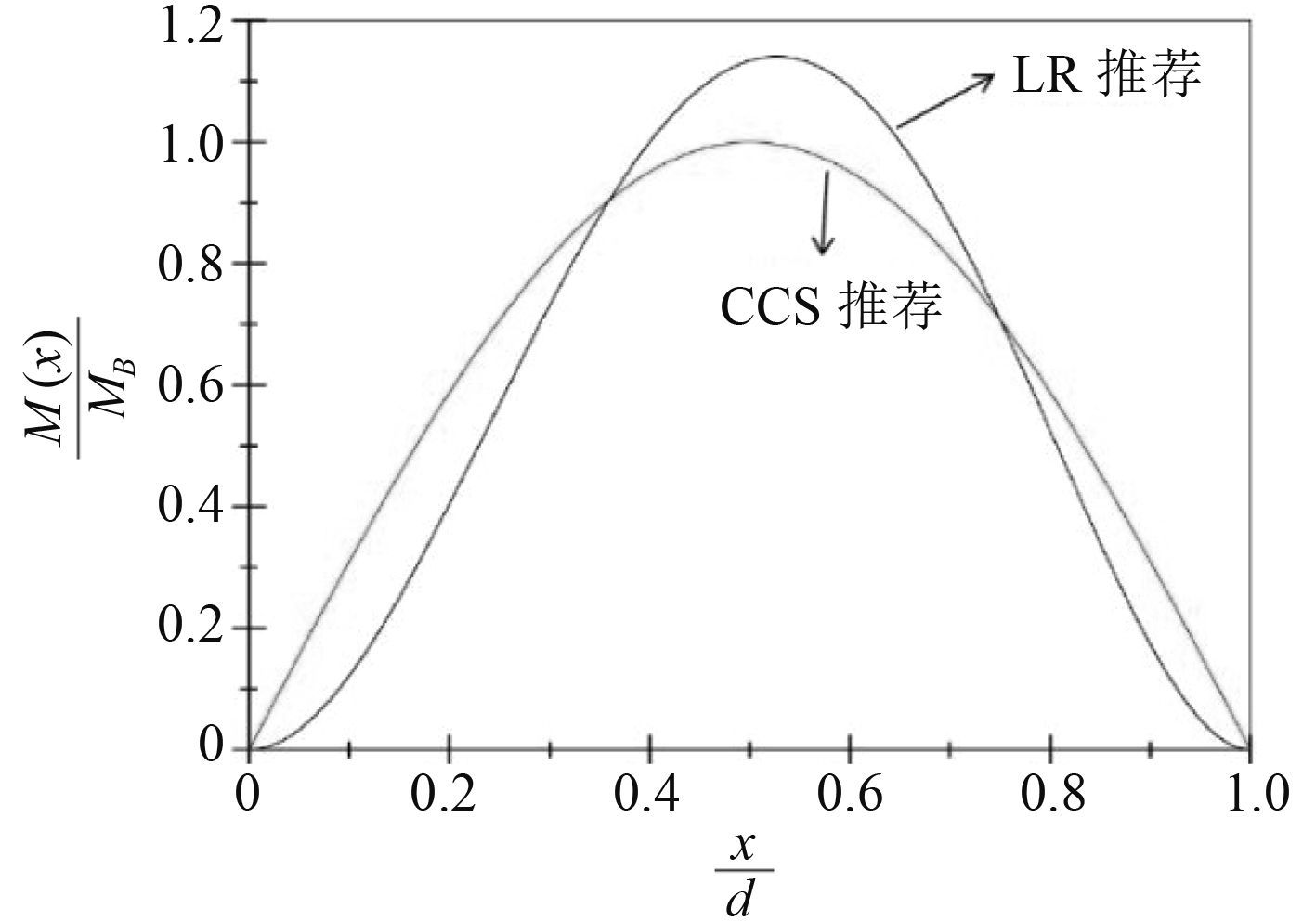图 1 总纵弯矩曲线分布对比图 Fig. 1 The comparison of longitudinal bending moment curves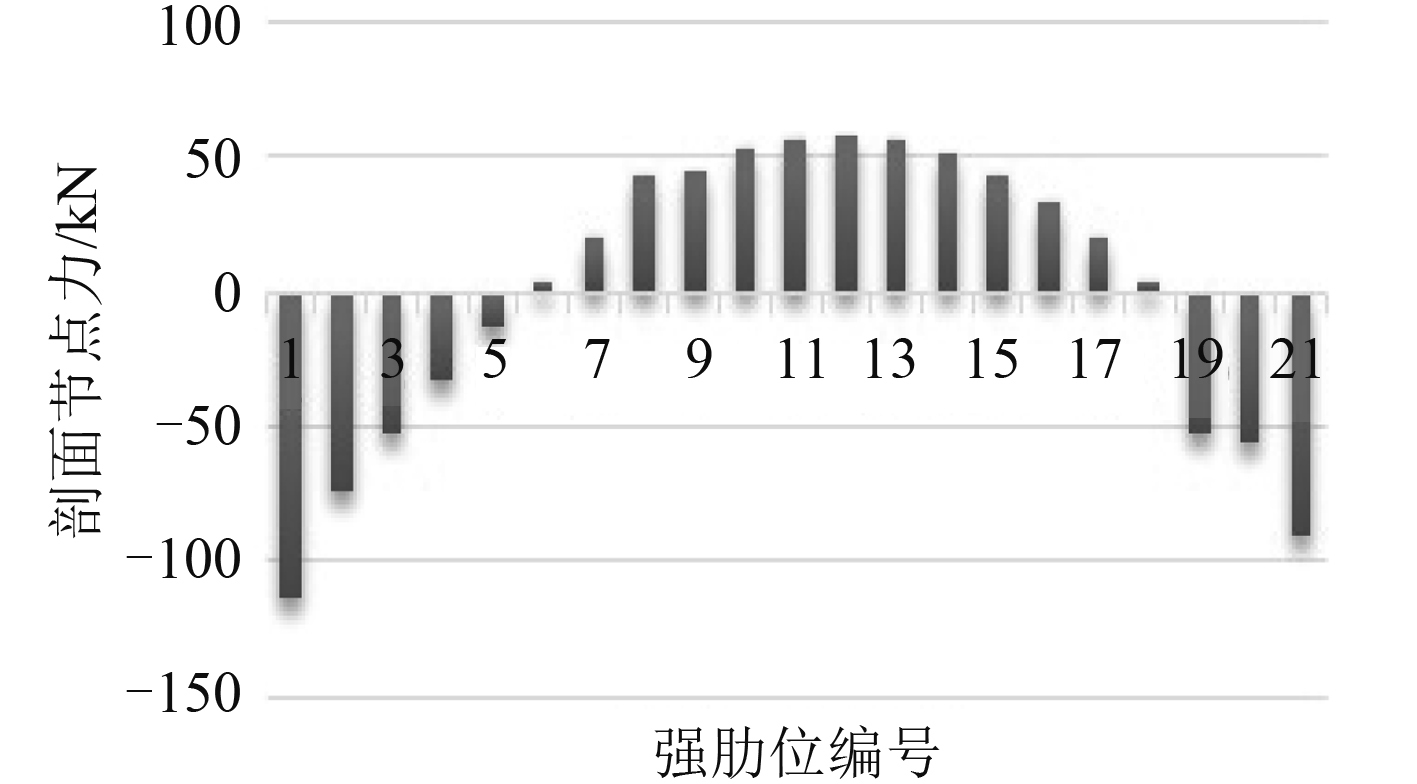图 2 中拱时不同横剖面等效节点载荷（CCS 推荐方法） Fig. 2 Equivalent nodal forces of different transverse section when hogging（recommended by CCS）表 1 全船不同肋位分段应力比较 Tab.1 The comparison of stresses in different fragments of the whole ship
2.2 总扭矩的施加位置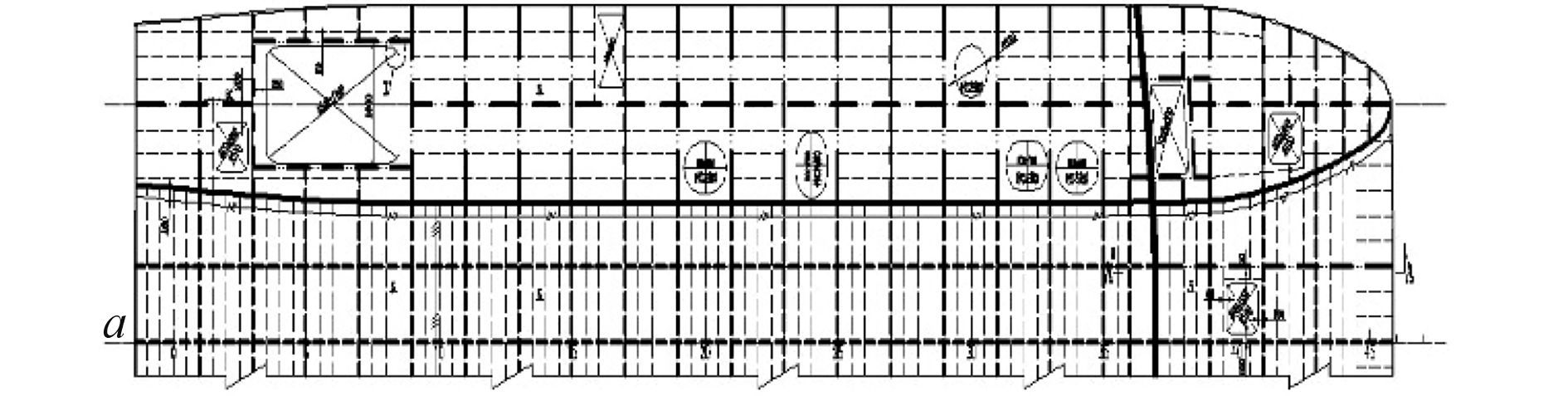图 3 主甲板结构图 Fig. 3 The structure plan of main deck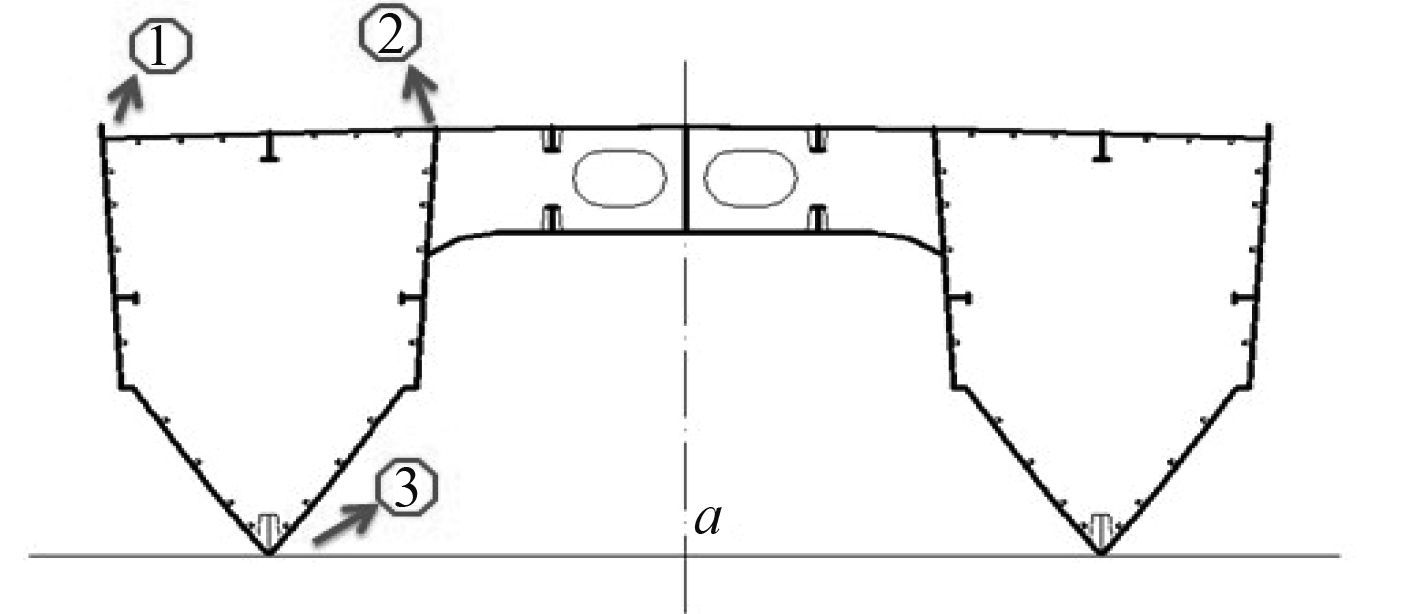图 4 总扭矩应力加载位置 Fig. 4 The loading locations of total torque表 2 三种方案应力结果 Tab.2 The stress results of three plans

3 全船结构强度分析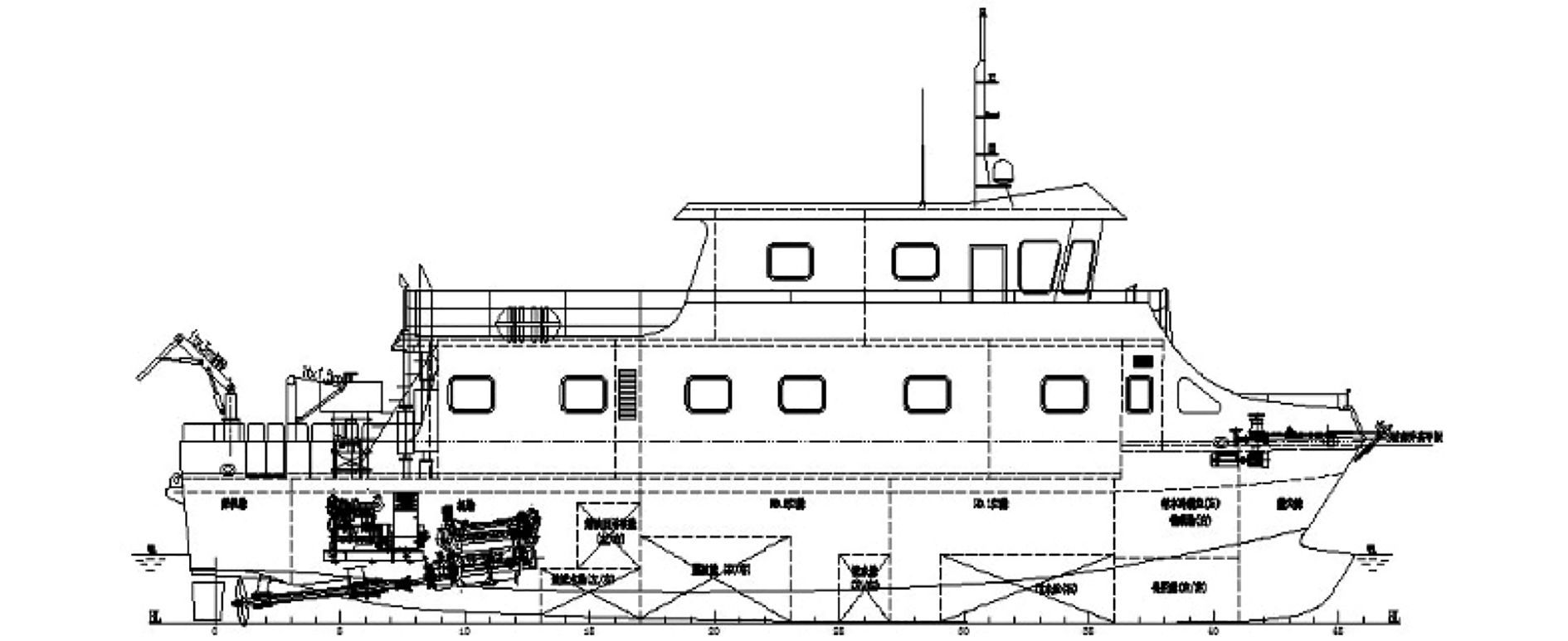图 5 总布置侧视图 Fig. 5 The side view of general arrangement表 3 计算工况 Tab.3 Load cases表 4 双体船全船板单元应力计算结果 Tab.4 The results of plate element stresses of the whole catamaran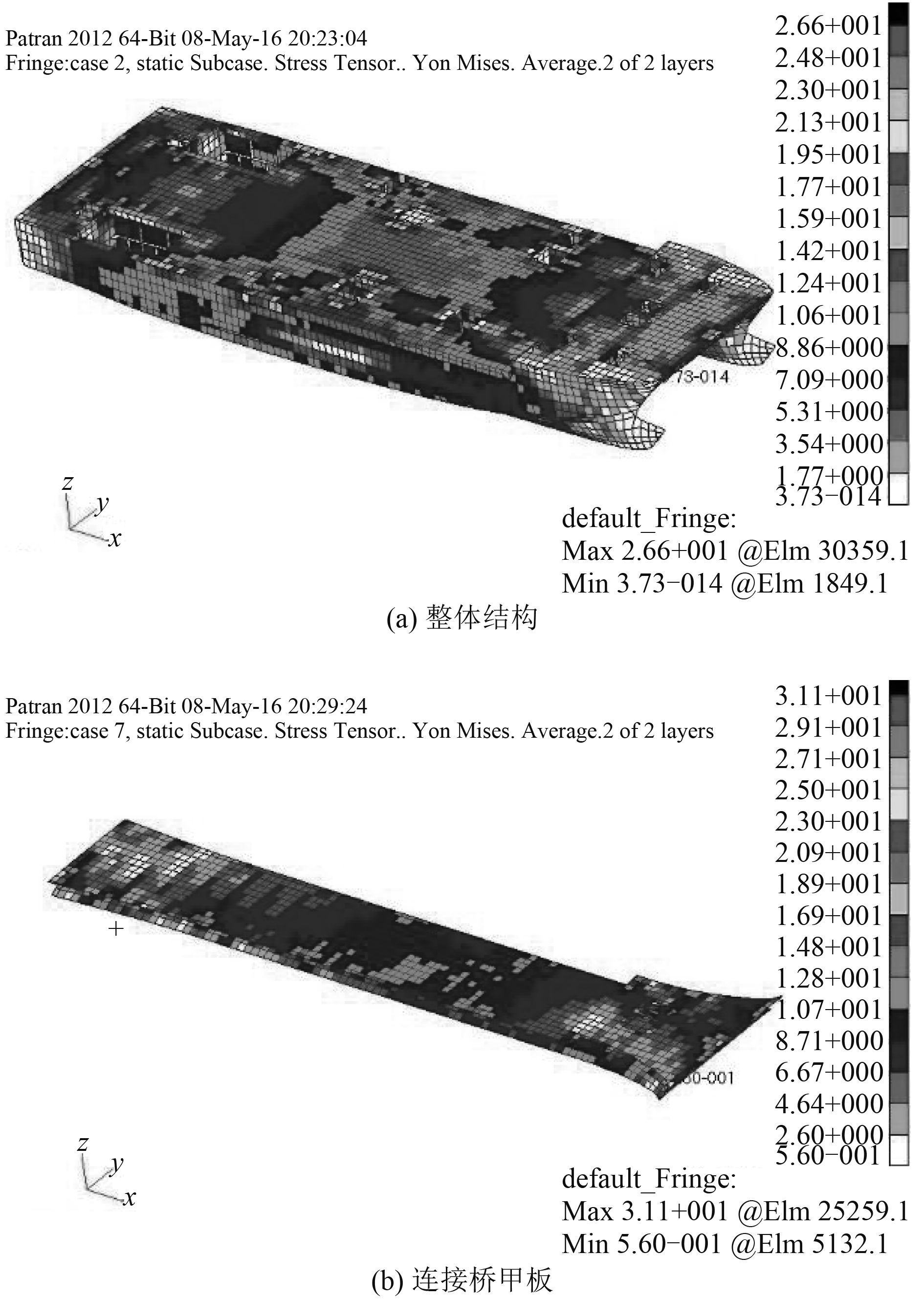图 6 Von-Mise 应力云图 Fig. 6 The Von-Mise stress nephogram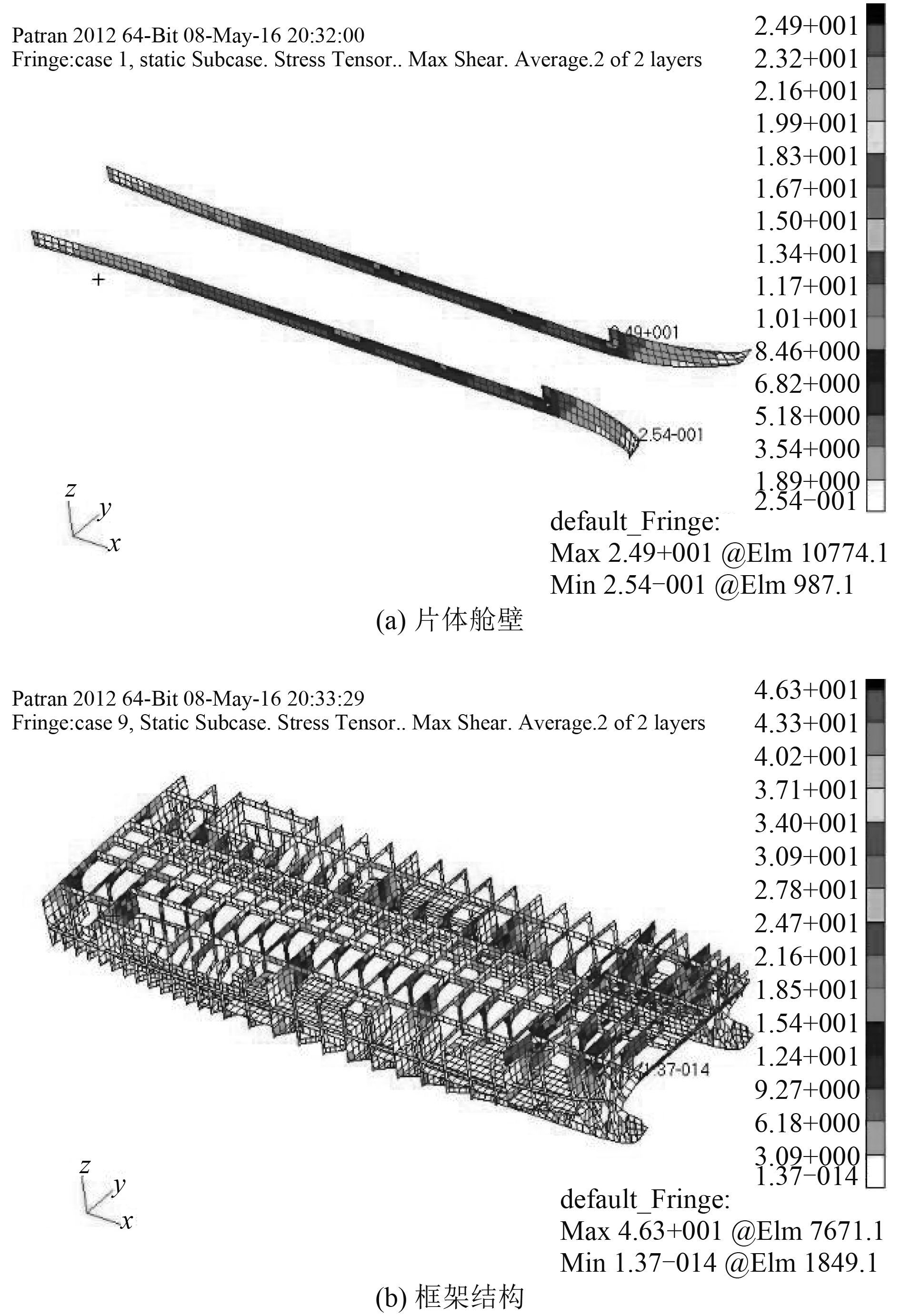图 7 Max Shear 应力云图 Fig. 7 The max shear stress nephogram

4 结 语

  崔连琼, 吴诗寒, 禚茜萍, 等. 非高速双体船波浪载荷直接计算方法研究[J]. 中国水运, 2015, 15 (8): 21–23. CUI Lian-qiong, WU Shi-han, ZHUO Qian-ping, et al. The research of direct calculation method for wave loading of non-high-speed catamaran[J]. China Water Transport, 2015, 15 (8): 21–23.  管义锋, 黄涣青, 谷家扬, 等. 双体铝合金高速客船强强度有限元分析研究[J]. 船舶工程, 2011, 33 (6): 14–17.  中国船级社. 海上高速船入级与建造规范[M]. 北京: 人民交通出版社, 2012.  江雪云, 冯国庆, 张清越, 等. 小水线面双体船波浪载荷预报研究[J]. 船舶工程, 2015, 37 .  徐永超, 夏利娟. 半潜驳改装海上风机吊装船结构强度评估[J]. 舰船科学技术, 2014, 36 (5): 11–14. XU Yong-chao, XIA Li-juan. Research on structural strength evaluation of wind turbine installation vessel retrofitted from semi-submersible barge[J]. Ship science and Technology, 2014, 36 (5): 11–14.  胡犇, 许晟, 梅国辉, 等. 基于Patran的高速小水线面双体船有限元结构强度分析[J]. 舰船科学技术, 2011, 33 (10): 46–49. HU Ben, XU Sheng, MEI Guo-hui, et al. Strength analysis of overall ship FEM model based on design wave approach[J]. Ship Science and Technology, 2011, 33 (10): 46–49.  林吉如, 石理国, 尤国红, 等. 小水面双体船波浪设计载荷估算方法[J]. 中国造船, 2008, 49 (3): 104–111.  LR. Guidance on direct calculations for primary structure of passenger ships[S]. 2004.  中国船级社. 海上高速船入级与建造规范[M]. 北京: 人民交通出版社, 2005.  郑杰, 谢伟, 骆伟, 等. 穿浪双体船横向强度与扭转强度的有限元计算[J]. 中国舰船研究, 2010, 5 (1): 14–18. ZHENG Jie, XIE Wei, LUO Wei, et al. Finite element analysis on the transverse and torsion strength of a wave piercing catamaran[J]. Chinese Journal of Ship Research, 2010, 5 (1): 14–18.